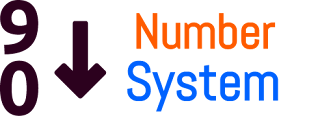## Elitmus Number System Questions-1 | Elitmus Papers

•12:38:00 AM
•If you read Number System in Quantitative section. Tutorial Diary presents Elitmus Quantitative Ability - Number System important and previous questions with answers and solutions.

Elitmus Number System Questions-1 | Elitmus PapersElitmus Number System Questions-1 | Elitmus Papers

Question: What is the remainder of 18!/23?
(a) 1
(b) 2
(c) 3
(d) 4

Solution:
Let rem[18!/23]=r
Sincw, Wilson's Theorem says, rem[(p-2)!/p]=1
rem[21!/23]=1
rem[21*20*19*18!/23]=1
rem[(-2)(-3)(-4)*r/23]=1
rem[-24r/23]=1
24r=-23K+1 ... k=-1
r=23+1/24
r=1

Question: If N= 1!+2!+3!.....+10!. Then, what will be the last digit of N^N?
(a) 3
(b) 7
(c) 1
(d) 4

Solution:
1! + 2! + 3! + 4! + 5! + 6! +7! +8! +9! +10!
1 + 2 + 6 + 24 + 120+........(from here all the last digits are zeros)................
The last digit of above sum is 3
So if we cube 3 ==> (3^3)=27

Question: A set M contains element all even number between 1 and 23 and all odd numbers 24 and 100. if all the elements of the set multiplied than how many trailing 0 , resulting number will contains?
(a) 10
(b) 12
(c) 9
(d) 13

Solution:
m=(2,4,6,8,10,12,14,16,18,20,22,25,27,29,31,33,35,37,39,41,43,45,47,49,51,53,55,57,59,61,63,65,67,69,71,73,75,77,79,81,83,85,87,89,91,93,95,97,99)
question asked how many trailing 0
there are two condition
1st if we multiply 2*5=10
end with =0
if we count 5 in set m we get 12
if we count 2 in set m we get more than 12
so total no end with 0 equal to 12

Question: if p is number greater than 99 ,e is the probability of divisiblity of any even whole number p, and O is the probablity of any odd whole number divisible by p , w is probablity of any whole number divisible by p.
(a) w=2e
(b) e=w/2
(c) o=w/2
(d) either (b) or (c)

Question: how many no. will be possible between 100 to 500 such that the sum of two digit is equal to the third(example -312, 2+1=3)??
a)12
b)24
c)66
d)72

Solution: for 123 total permutation 123,132,213,231,312,321
123=6
134=6
145=4 because in 6 case two case 541>500 and 514>500
156=2 because in 6 case four case 651>500 and 615>500 and 516 ,561
167=2
178=2
189=2

235=4
246=4
257=2
268=2
279=2

347=4
358=2
369=2
total=46
and
101,110
202,220
303,330
404,440
total=8
121,221,112
242,224,422
336,363
448,484
total=10

Total no of case 46+8+10=66

Question: find remainder of (9^1+9^2+.........+9^n)/6
n is multiple of 11.
(a) 0
(b) 5
(c) 3
(d) can not be determine

Solution:
Since:
9^(1+2+3+11n)/6
=>9^(11n(11n+1)/2)/6 (suppose it is 11)
=>9^11(11+1)/6*2=3(ans)
=>9^22(22+1)/6*2=3(ans)

Question: the square of two digit number(ab) divided by half of itself resultant added by 18 then divided by 2 then we get reverse of original number(ba). how many combination of ab exist
(a) 9
(b) 8
(c) 7
(d) 6

Solution:
(((10a+b)^2)/(10a+b/2)+18)/2=10b+a.
Simplify the above equation.
The final equation will be a-b=-1
There will be 8 combinations of a &b which will satisfy this equation.
i.e.
a=1,b=2
a=2,b=3
a=3,b=4
a=4,b=5
a=5,b=6
a=6,b=7
a=7,b=8
a=8,b=9

Question: Find the 100th place digit of a the greatest number forming from digits 1 to 9 without repeatation and number passes the divisibility test of 11 means difference of sum of alternative digits is 0 or divisible by 11.
(a) 1
(b) 2
(c) 3
(d) 4

Solution: The 100th place digit would be 4.
Since the largest number which is divisible by 11 having digits 1 to 9 is 978653412,
the difference of the sum of the digits at even place and odd place is 11, which is a multiple of 11.

Hope you like this, share it with your friends to help them.